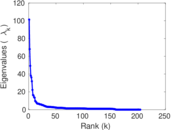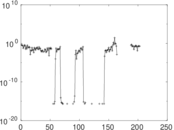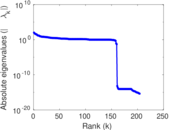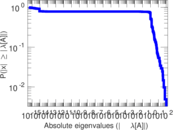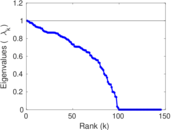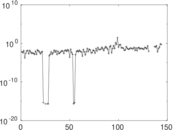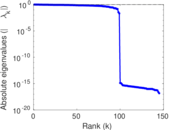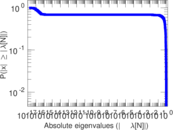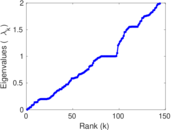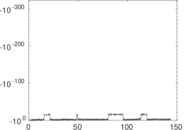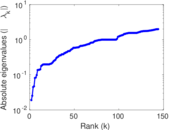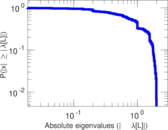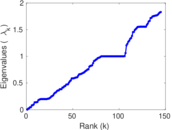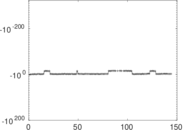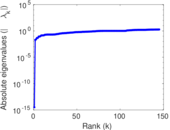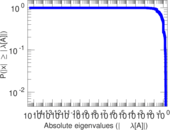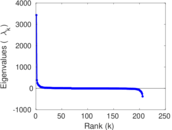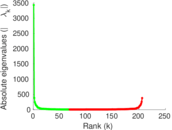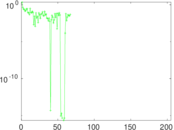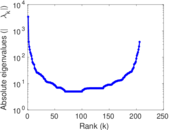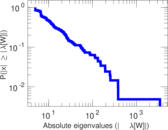# Wikiquote edits (li)

This is the bipartite edit network of the Limburgish Wikisource. It contains users and pages from the Limburgish Wikisource, connected by edit events. Each edge represents an edit. The dataset includes the timestamp of each edit.

 Code `qli` Internal name `edit-liwikisource` Name Wikiquote edits (li) Data source http://dumps.wikimedia.org/ AvailabilityDataset is available for download Consistency checkDataset passed all tests Category Authorship network Dataset timestamp 2017-10-20 Node meaning User, article Edge meaning Edit Network formatBipartite, undirected Edge typeUnweighted, multiple edges Temporal dataEdges are annotated with timestamps

## Statistics

 Size n = 3,437 Left size n1 = 206 Right size n2 = 3,231 Volume m = 6,143 Unique edge count m̿ = 4,386 Wedge count s = 2,105,255 Claw count z = 954,862,506 Cross count x = 342,990,013,422 Square count q = 134,569 4-Tour count T4 = 9,519,328 Maximum degree dmax = 2,412 Maximum left degree d1max = 2,412 Maximum right degree d2max = 168 Average degree d = 3.574 63 Average left degree d1 = 29.820 4 Average right degree d2 = 1.901 27 Fill p = 0.006 589 68 Average edge multiplicity m̃ = 1.400 59 Size of LCC N = 3,224 Diameter δ = 11 50-Percentile effective diameter δ0.5 = 3.183 87 90-Percentile effective diameter δ0.9 = 4.627 11 Median distance δM = 4 Mean distance δm = 3.415 86 Gini coefficient G = 0.698 938 Balanced inequality ratio P = 0.215 530 Left balanced inequality ratio P1 = 0.084 812 0 Right balanced inequality ratio P2 = 0.343 155 Relative edge distribution entropy Her = 0.710 347 Power law exponent γ = 5.735 56 Tail power law exponent γt = 2.771 00 Tail power law exponent with p γ3 = 2.771 00 p-value p = 0.000 00 Left tail power law exponent with p γ3,1 = 1.821 00 Left p-value p1 = 0.411 000 Right tail power law exponent with p γ3,2 = 2.941 00 Right p-value p2 = 0.000 00 Degree assortativity ρ = −0.289 975 Degree assortativity p-value pρ = 1.008 41 × 10−85 Spectral norm α = 101.346 Algebraic connectivity a = 0.018 942 1 Spectral separation |λ1[A] / λ2[A]| = 1.488 73 Controllability C = 3,105 Relative controllability Cr = 0.906 569

## Plots

### Fruchterman–Reingold graph drawing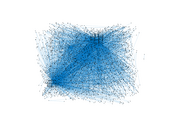### Degree distribution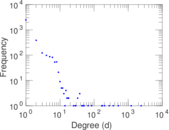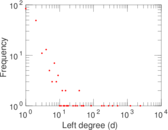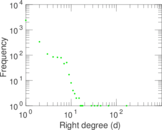### Cumulative degree distribution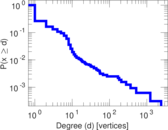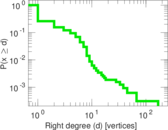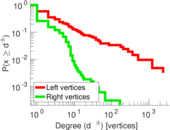### Lorenz curve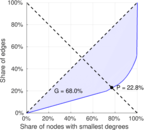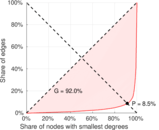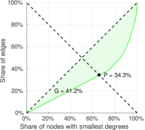### Spectral distribution of the adjacency matrix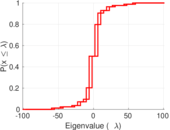### Spectral distribution of the normalized adjacency matrix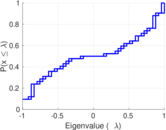### Spectral distribution of the Laplacian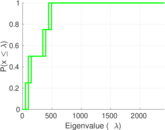### Spectral graph drawing based on the adjacency matrix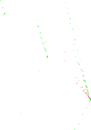### Spectral graph drawing based on the Laplacian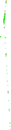### Spectral graph drawing based on the normalized adjacency matrix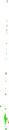### Degree assortativity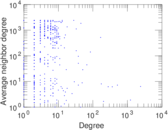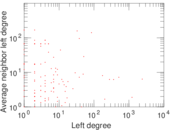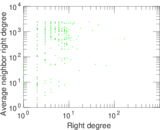### Zipf plot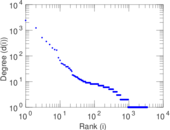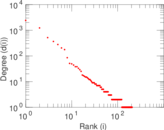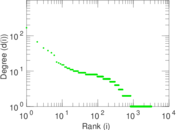### Hop distribution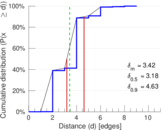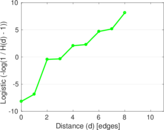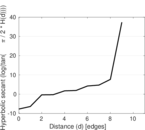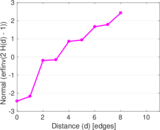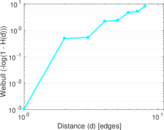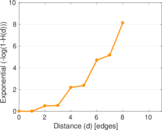### Double Laplacian graph drawing### Delaunay graph drawing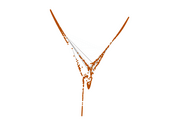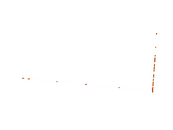### Edge weight/multiplicity distribution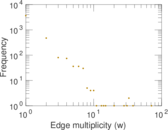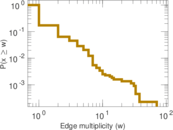### Temporal distribution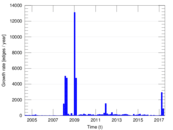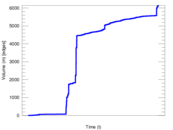### Temporal hop distribution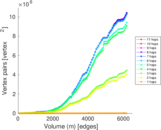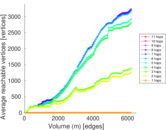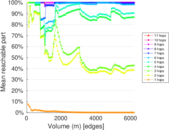### Diameter/density evolution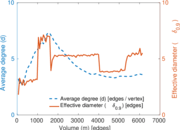### Matrix decompositions plots